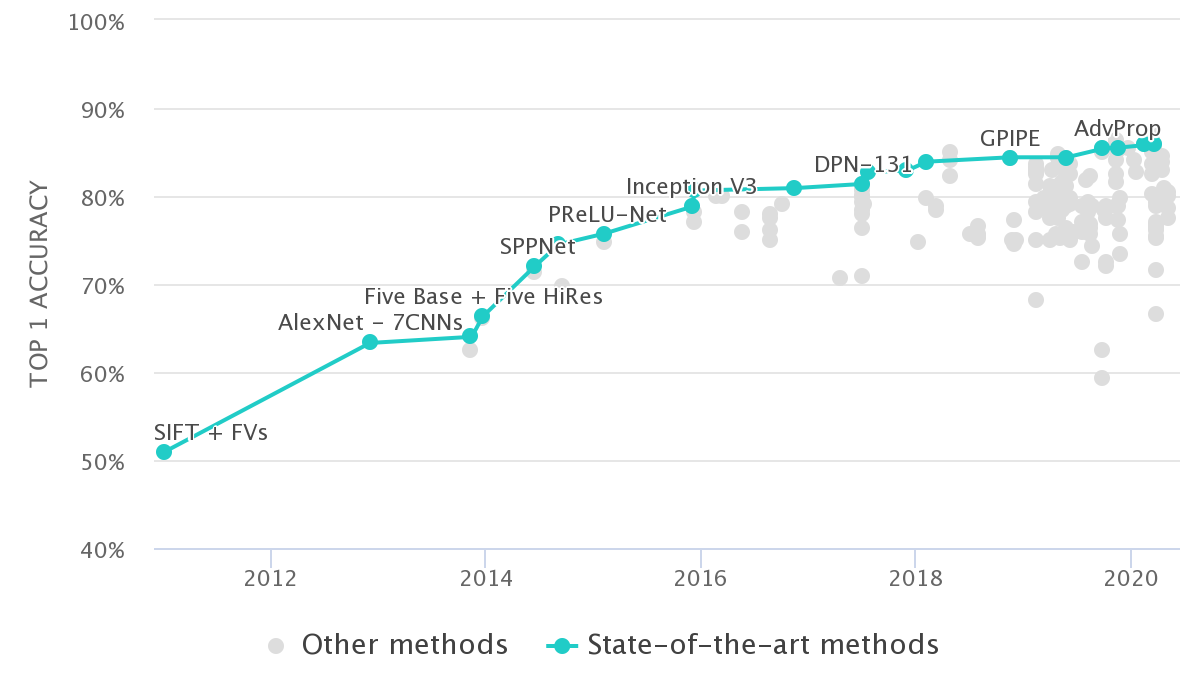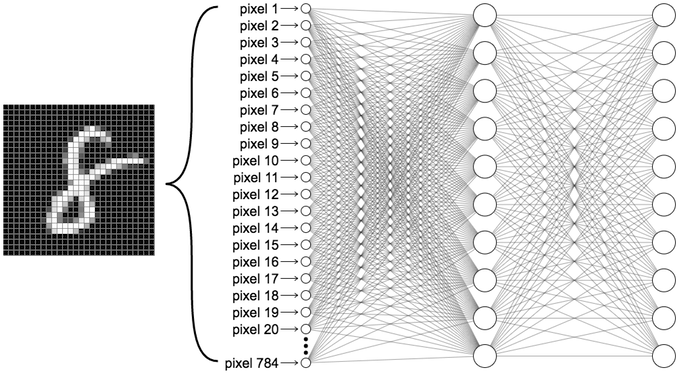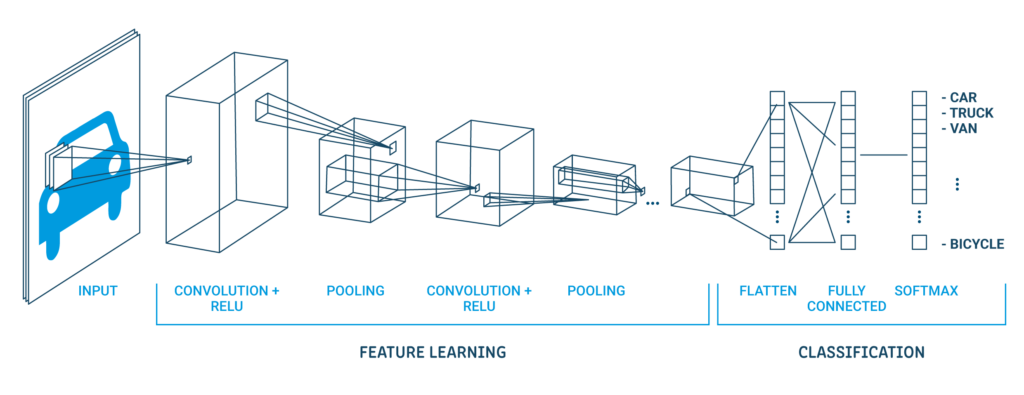## Introduction

In this blogpost I will be explaining the difference between Model centric and Data centric Machine Learning. Here Machine Learning refers to branch of AI which learns to perform tasks by learning a model from a labelled dataset. So it would include conventional ML methods and Deep Learning. In machine learning we have a dataset and a model with its associated parameters. A model which gives relationship between input and output pairs. This model could also provide predictions for datapoints not seen in the training set. This blogpost aims to address a question: If I have a certain accuracy with a given model and given dataset on a task. Can I improve the accuracy of my model by getting more data (data-centric) or improving the model (model-centric)? Model centric approach refers to almost all practices to improve a ML model performance that don’t involve gathering a larger dataset or using any external dataset that is keeping the dataset fixed. While data centric approach refers to improving model performance by incorporating bigger or external datasets. Performance here strictly refers to accuracy of a given model on unseen data, what you called generalization error.

## Priors and Data

In order to get a better understanding of foundations between the two approaches, I would like to explain how priors and data shape models in machine learning. It is a standard practice to train a very complex model such as neural networks with lots of data. Alternatively train an appropriate model with right assumptions that captures the relationship between input and output variables to a large degree. An example of second approach could be a logistic regression or Linear Regression model trained on data that exhibits close to linear relationship between input and output variables. The model works well if the relationship between the input and target variables are linear. Even if its not linear, it is upto the ML Practitioner to design features that are linearly related or any other feature transformation to fit a given models assumptions. This approach requires very less data because the model structure captures the relationship of the data. Or the ML practitioner is using their understanding of the problem to design relevant features that fit the model. Both of these involve some kind of prior knowledge of the dataset. While, priors allow us to use lesser data they can be very difficult to design. It would require the practitioner to make a lot of assumptions of the complex world. On the contrary in the first case discussed above, powerful models likes neural networks don’t require much understanding of the task or dataset. Users could directly fit the model on a very large representative labelled dataset and get a decent enough accuracy. But, can require lots of samples and compute power of data to learn the task. To make the difference more clear lets take a simple example of detecting the wheel of a car. A simple model is using our common sense reasoning in mathematical form. Using image processing to detect rubber, rims, road and other attributes that define a wheel. But, then there are so many possible combinations of these attributes which can vary in different conditions. Identifying priors to accommodate all the possible conditions might be a tedious process. Another way to solve the same problem is to gather images of 1000’s of wheels and have a complex model learn from it. This phenomenon is statistically explained by the concept of bias-variance tradeoff.

## Model Centric ML

Model centric ML is the practice of keeping data constant and changing the ML code to improve performance of the model. Before I explain model centric AI, I would like to mention models don’t just refer to mathematical model(neural network, SVM, logistic regression,etc) but refers to other practices with respect to model that make it easier to learn. Practices such as hyper parameters, loss function, training practices, representations and augmentations. Generating Synthetic dataset, augmentations and learning representations count as model centric ML. Lets simply redefine model as ML code (could simply mean a Pytorch or Tensorflow code) that runs on a fixed input dataset. It's an approach that takes a lot longer to improve performance. It requires deep expertise and understanding of practitioner of ML models and the given problem. Model centric ML is more of a research approach.Credits: Papers with Code

To get an idea of pace of improvement you can have a look at Imagenet benchmarks over the years. Imagenet is a competition where people train models on a large fixed dataset of images of 1000 different categories and report their accuracies and methods. Imagenet is a very model centric competition. That is contestants are not allowed to use external datasets. But, must solely increase accuracies by improving their model. You can see over the years that the improvements of accuracies are very slow. Almost all the contestants have used their prior knowledge of the dataset or of the neural network itself to improve performance. So it incorporates prior knowledge of some or the other kind. Model centric ML is mostly practiced in academic research and competitions. To some extent in industry, but not primarily.

## Data Centric ML

Data centric ML is the practice of keeping model code constant and augmenting dataset to improve performance of the model. Like I mention in the priors vs data section, complex models learn better with larger data. Data centric approach is a lot easier and faster as compared to model centric ML. But, is expensive. It requires gathering large amounts of data. Maybe even effort into annotating them. Further, requires more infrastructure for storing and managing dataset. Complex models usually have lot more parameters and require more hardware infrastructure for training the models. It is a common for industries to primarily focus on data centric ML as opposed to model centric ML. With significant emphasis of data, most effort in data centric ML is into storing, managing and cleaning large datasets. Although you can fit a model to get some decent accuracy. In data centric approach, understanding what data is relevant for a given task is still important. The dataset determines the model quality. I explain this in detail in my other blogpost titled "Deep Learning in Practice-Be The algorithm".

## What is a better approach?

It's not a dichotomy. They are both beneficial and used at some proportions in the industry. Data centric ML is beneficial on a short term. While, Model centric ML is much more beneficial on a long term. In cases, where there isn’t enough data or infrastructure model centric ML becomes the way to do. In fact data centric ML is a result of model centric ML. More complex models have given us the flexibility to learn a task from almost any data source allowing data centric ML to be a possibility. Academic research is focused primarily on model centric ML.Having described model centric and data centric, I will give an example that unifies both themes and how they go hand in hand. Until, 1999 MLPs were the way to go about training models for image classification tasks. Until, Yann Lecunn came up with Convolutional Neural Network (CNN’s). It improved the accuracy of MNIST by a few % with much lesser parameters. MLP’s could do fine with a simple dataset like MNIST. CNN’s were different because they had encoded a prior that features that existed could be anywhere in the image and not any specific location, a property called translation invariance. Earlier MLP’s required every possible location of feature requiring it to be trained on a lot more data.

A CNN trained on the first image could generalise to other two images. But, an MLP would have to be trained on all the three images and a combination of other positions. CNN’s dint just help improve accuracy of ML algorithms on Computer vision tasks, but also reduced the compute and number of parameters required, making it more scalable to train models for various CV tasks. This is an example of how Model centric AI could reduce the effort of Data centric ML.Multi Layer Perceptron (MLP)Convolutional Neural Network (CNN)

To summarize if you have a lot of labelled data, compute infrastructure and short-term targets use data centric ML. Else use model-centric or a combination of both.